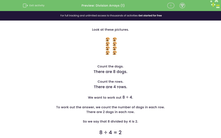# Use Division Arrays

In this worksheet, students will match pictorial arrays to division statements.Key stage:  KS 1

Curriculum topic:   Number: Multiplication and Division

Curriculum subtopic:   Solve Multiplication/Division Problems

Popular topics:   Multiplication worksheets, Division worksheets

Difficulty level:#### Worksheet Overview

Take a look at these pictures.Can you count the dogs?

There are 8 dogs.

Now let's count the rows.

There are 4 rows.

We want to find out what is 8 shared between 4.

We write this as  8 ÷ 4.

To work out the answer, we count the number of dogs in each row.

There are 2 dogs in each row.

So we say that 8 divided by 4 is 2.

8 ÷ 4 = 2

Does that make sense?Let's try some questions now.

### What is EdPlace?

We're your National Curriculum aligned online education content provider helping each child succeed in English, maths and science from year 1 to GCSE. With an EdPlace account you’ll be able to track and measure progress, helping each child achieve their best. We build confidence and attainment by personalising each child’s learning at a level that suits them.

Get started